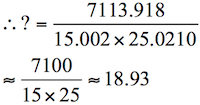# Sample Aptitude Questions of HCL Technologies

Aptitude section forms a part of almost every placement test. In the case of HCL written paper, there are 15 questions from various topics of quantitative/ numerical aptitude. These questions are based on number system, time & work, profit & loss, geometry, mixtures, probability, permutation & combination, and similar other topics. Here are some common practice questions for the aptitude section of HCL test:
###### HCL Aptitude Questions
1. A basket contains 3 blue, 5 black and 3 red balls. If 3 balls are drawn at random what is the probability that all are black?
1. 2/11
2. 1/11
3. 3/11
4. 8/33
5. None of these
Ways of selecting 3 black balls out of 5 - 5C3
Total ways of selecting 3 balls - 11C3The required probability =
5C3 / 11C3 = 10/165 = 2/33
DIRECTIONS for questions 2-3: What should come in place of question mark (?) in the following number/alphabetic series?
1. 17 9 10 ? 35 90
1. 21
2. 27.5
3. 19
4. 16.5
5. None of these
17×0.5+0.5=9
9×1+1=10
10×1.5+1.5=16.5
16.5×2+2=35
35×2.5+2.5=90
Hence, the question mark(?) should be replaced by 16.5
2. 3 20 78 334 1696 ?
1. 8410
2. 9836
3. 10206
4. 1150
5. None of these
3×2+14=20
20×3+18=78
78×4+22=334
334×5+26=1696
1696×6+30=10206
Hence, the question mark should be replaced by 10206
1. The magnitude of the area of a circle is seven times that of its circumference. What is the circumference (in units) of the circle?
1. 616
2. 132
3. 88
4. Can't be determined
5. None of these
We have πr2 = 7 x 2πr
∴ r = 14
∴ circumference = 2πr = 2 x (22/7) * 14 = 88
2. 15.002 × ? × 25.0210 = 7113.018
1. 19
2. 26
3. 11
4. 31
5. 35
15.002 × ? × 25.0210 = 7113.0183. A mixture of 45 liters of spirit and water contains 20% of water in it. How much water must be added to make the water 25% in the new mixture?
1. 5 liters
2. 3 liters
3. 4 liters
4. 6 liters
5. None of these
Quantity of water in the mixture = (20/100) x 45 = 9 L
Let x litres of water must be added to make the water 25 % in the solution
Therefore, [(9+x)/(45+x)] x 100 = 25
⇒ 36 + 4x = 45 + x
⇒ 3x = 9
⇒ x = 3 litres
4. The sum of four consecutive integers is 102. Find the product of the extremes:
1. 548
2. 684
3. 648
4. 578
5. None of these
a,a+1,a+2,a+3 , let these be the numbers.
Sum=102⇒4a+6=102⇒4a=96⇒a=24
Hence extremes=24 , 27
24X27=648
5. A bottle contains 3/4 of milk and the rest water. How much of the mixture must be taken away and replaced by equal quantity of water so that the mixture has half milk and half water?
1. 25%
2. 3312%
3. 45%
4. 50%
5. None of these
Let the total quantity of mixture = 1 liter.
Now supposing x liters of mixture is withdrawn which contains 3/4x milk and rest water.
So, according to the question 3/4 -3/4x =1/2.
Solving x = 1/3 which means 1/3 of mixture is to be withdrawn to serve the purpose.
6. Krishan has some hens and some cows. If the total number of animal heads are 59 and the total number of feet are 190, how many cows does Krishan have?
1. 36
2. 32
3. 23
4. 20
5. Can't be determined
Suppose he has x cows and y hens.
So x + y = 59 and 4x + 2y = 190.
Solving these two equations, we get x = 36.
So option A is the answer
7. The height of a room is 5 metres and its length is twice its breadth. If 240 metres of paper of breadth 50 cm are required for papering its walls, find the area of the floor.
1. 128 m2
2. 64 m2
3. 320 m2
4. 32 m2
5. None of these